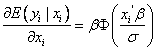# st: Re: Re:Re:xttobit

 From "Marina.Balboa" To Subject st: Re: Re:Re:xttobit Date Thu, 17 Jul 2003 19:03:03 +0200

```Dear statalist,

I would like to be sure about just one thing. In the Tobit model, you could
be interested in the following marginal effects:
1)

2)

3)

where y* is the latent variable and y is the censored variable. I just have
two questions:

i) Are the coefficients that Stata gives in xttobit  the ones numbered as 1?
(the marginal effect of the latent variable). I suppose it is this way. If
this were true, I could calculate 2 and 3 from 1 by hand.

ii) Is there any other way to obtain 2 and 3 directly without doing by had?
(mfx compute?)

Thank you to all,

> Thanks Scott!

> > Hello!
> >
> > I would be so happy if someone could help me. I am estimating a tobit
> panel data model and I would like to know if it is possible to calculate
> marginal effects in xttobit.
> >
> >
> > Paula Garcia
> >
>
> Yes,  take a look at -mfx compute-
>
> With it you can calculate the margin effects of the probability of being
> uncensored, conditional on being uncensored, and the unconditional
expected
> value.
>
>
> Hope this helps,
> Scott
>
```